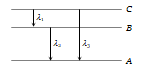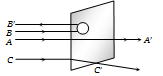Every series of hydrogen spectrum has an upper and lower limit in wavelength. The spectral series which has an upper limit of wavelength equal to 18752 Å is
(a) Balmer series                (b) Lyman series
(c) Paschen series               (d) Pfund series

(Rydberg constant R = $1.097×{10}^{7}$ per metre)

Concept Questions :-

Spectral series
High Yielding Test Series + Question Bank - NEET 2020

Difficulty Level:

The kinetic energy of the electron in an orbit of radius r in hydrogen atom is (e = electronic charge)
(a) $\frac{{\mathrm{e}}^{2}}{{\mathrm{r}}^{2}}$                (b) $\frac{{\mathrm{e}}^{2}}{2\mathrm{r}}$
(c) $\frac{{\mathrm{e}}^{2}}{\mathrm{r}}$                (d) $\frac{{\mathrm{e}}^{2}}{2{\mathrm{r}}^{2}}$

Concept Questions :-

Bohr's model of atom
High Yielding Test Series + Question Bank - NEET 2020

Difficulty Level:

Energy levels A, B, C of a certain atom corresponding to increasing values of energy i.e. ${\mathrm{E}}_{\mathrm{A}}<{\mathrm{E}}_{\mathrm{B}}<{\mathrm{E}}_{\mathrm{C}}$. If  are the wavelengths of radiations corresponding to the transitions C to B, B to A and C to A respectively, which of the following statements is correct(a) ${\mathrm{\lambda }}_{3}={\mathrm{\lambda }}_{1}+{\mathrm{\lambda }}_{2}$                      (b) ${\mathrm{\lambda }}_{3}=\frac{{\mathrm{\lambda }}_{1}{\mathrm{\lambda }}_{2}}{{\mathrm{\lambda }}_{1}+{\mathrm{\lambda }}_{2}}$
(c) ${\mathrm{\lambda }}_{1}+{\mathrm{\lambda }}_{2}+{\mathrm{\lambda }}_{3}=0$                 (d) ${\mathrm{\lambda }}_{3}^{2}={\mathrm{\lambda }}_{1}^{2}+{\mathrm{\lambda }}_{2}^{2}$

Concept Questions :-

Spectral series
High Yielding Test Series + Question Bank - NEET 2020

Difficulty Level:

The angular momentum of electron in nth orbit is given by

(a) nh                 (b) $\frac{\mathrm{h}}{2\mathrm{\pi n}}$
(c) $\mathrm{n}\frac{\mathrm{h}}{2\mathrm{\pi }}$             (d) ${\mathrm{n}}^{2}\frac{\mathrm{h}}{2\mathrm{\pi }}$

Concept Questions :-

Bohr's model of atom
High Yielding Test Series + Question Bank - NEET 2020

Difficulty Level:

The ratio of the energies of the hydrogen atom in its first to second excited state is
(a) 1/ 4                    (b) 4/9
(c) 9/ 4                     (d) 4

Concept Questions :-

Bohr's model of atom
High Yielding Test Series + Question Bank - NEET 2020

Difficulty Level:

An electron jumps from the 4th orbit to the 2nd orbit of hydrogen atom. Given the Rydberg's constant R = . The frequency in Hz of the emitted radiation will be
(a) $\frac{3}{16}×{10}^{5}$           (b) $\frac{3}{16}×{10}^{15}$
(c) $\frac{9}{16}×{10}^{15}$          (d) $\frac{3}{4}×{10}^{15}$

Concept Questions :-

Bohr's model of atom
High Yielding Test Series + Question Bank - NEET 2020

Difficulty Level:

The ionisation potential of hydrogen atom is 13.6 volt. The energy required to remove an electron in the n = 2 state of the hydrogen atom is
(a) 27.2 eV                (b) 13.6 eV
(c) 6.8 eV                  (d) 3.4 eV

Concept Questions :-

Bohr's model of atom
High Yielding Test Series + Question Bank - NEET 2020

Difficulty Level:

If the wavelength of the first line of the Balmer series of hydrogen is 6561 $\stackrel{0}{\mathrm{A}}$, the wavelength of the second line of the series should be
(a) 13122 $\stackrel{0}{\mathrm{A}}$                  (b) 3280 $\stackrel{0}{\mathrm{A}}$
(c) 4860 $\stackrel{0}{\mathrm{A}}$                    (d) 2187 $\stackrel{0}{\mathrm{A}}$

Concept Questions :-

Spectral series
High Yielding Test Series + Question Bank - NEET 2020

Difficulty Level:

A beam of fast moving alpha particles were directed towards a thin film of gold. The parts A',B', and C' of the transmitted and reflected beams corresponding to the incident parts A, B and C of the beam, are shown in the adjoining diagram. The number of alpha particles in(a) B' will be minimum and in C' maximum
(b) A' will be maximum and in B' minimum
(c) A' will be minimum and in B' maximum
(d) C' will be minimum and in B' maximum

Concept Questions :-

Various atomic model
High Yielding Test Series + Question Bank - NEET 2020

Difficulty Level:

According to Bohr's theory the radius of electron in an orbit described by principal quantum number n and atomic number Z is proportional to
(a) ${\mathrm{Z}}^{2}{\mathrm{n}}^{2}$            (b) $\frac{{\mathrm{Z}}^{2}}{{\mathrm{n}}^{2}}$
(c) $\frac{{\mathrm{Z}}^{2}}{\mathrm{n}}$              (d) $\frac{{\mathrm{n}}^{2}}{\mathrm{Z}}$

Concept Questions :-

Bohr's model of atom